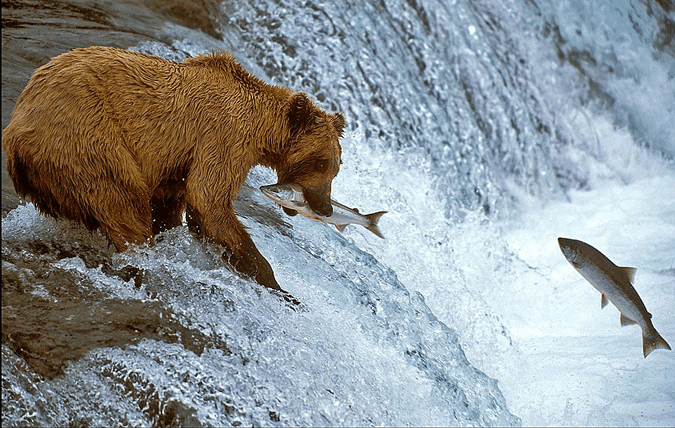# Random elements from a stream

sampling
stream
Published

June 25, 2011This is a classic trick when dealing with data streams. It shows how to draw a random element from a sequence of instances without knowing beforehand how long the sequence is and which symbols occur. Let us first assume that we knew the identities of all symbols. In this case finding a random symbol would be easy. All we require is that for each symbol $$s$$ we draw a random variable $$\xi_s \sim U[0,1]$$ from some distribution and subsequently we choose the symbol

$s^*=\mathop{\mathrm{argmin}}_s ~ \xi_s.$

Since each $$s$$ has equal probability of being associated with the smallest value $$\xi_s$$ it follows that the draw is uniformly random. The trouble with this is that we now need to store one floating point number per key $$s$$ and a method to look up its value, e.g. via a dictionary. This is just as tedious as recording the entire stream.

Here’s a better way. Assume that instead of requesting a random variable $$\xi_s$$ we simply compute the hash $$h(s)$$ of $$s$$ and we set

$s^*=\mathop{\mathrm{argmin}}_s ~ h(s).$

For a draw from the space of hash functions this again is uniform. The advantage is that we essentially determined all the random bits when selecting $$h$$ rather than at the time when we want to compute its value $$h(s)$$. The second advantage is that we can now simply keep track of what is the currently smallest value of $$h(s)$$ and update as we go along. We have the following algorithm:

INIT
hstar = MAXINT
n = 0
sstar = NONE
FOR ALL incoming s DO
IF h(s) = hstar:
n = n + 1
ELSE IF h(s) < hstar:
n = 1
hstar = h(s)
sstar = s
RETURN (sstar, n)

This algorithm will provide item counts for a random element of the sequence. If you want more than one sample, simply keep a list of the symbols with the $$k$$ smallest hash values and their associated counts. Such algorithms can be used to compute the variance or other moments of a sequence.

PS: The picture is from the Penticton Lakeside Resort where you can probably see such bears (no, I never visited).